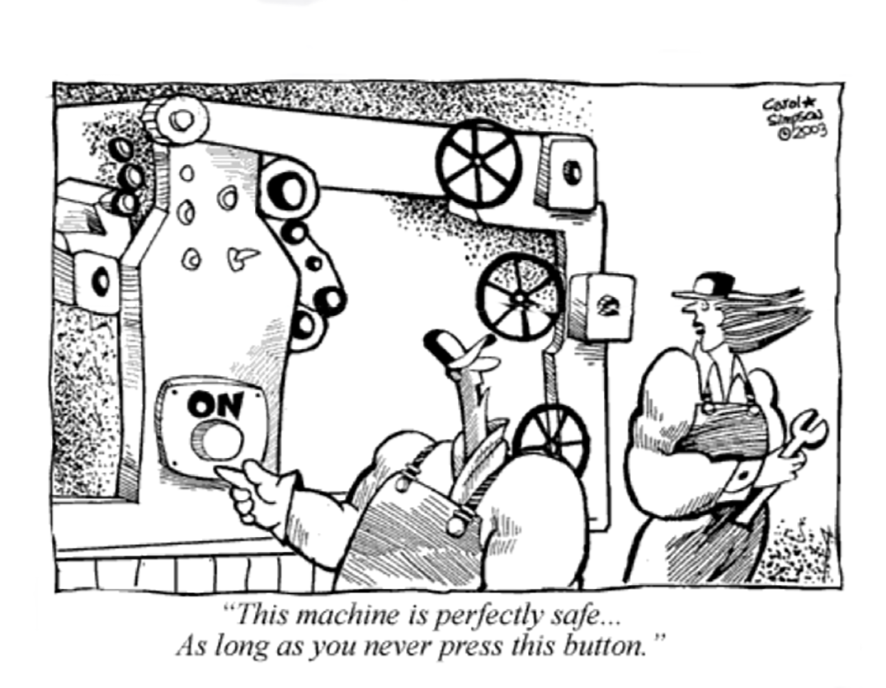When drafting a machinery Risk Assessment, one of the most controversial elements to be estimated is the probability of occurrence, and the main reason for such challenge is related to the meaning associated with the word “Probability”.
Often the term probability is confused with likelihood or predictability, which are derivatives of the concept of probability rather than a synonymous of it.

## Probability vs Likelihood

Generally, the term probability is used when describing a function of the outcome given a fixed parameter value. For example, if a coin is flipped 10 times and it is a fair coin1, what is the probability of it landing heads-up every time.
The likelihood is the probability that an event — that has already occurred — would yield a specific outcome.
First distinction: Probability refers to the occurrence of future events, while a likelihood refers to past events with known outcomes.
We could say that likelihood is the derivative of probability because it is similar to the difference between speed and distance.
If you integrate likelihood over an interval, you get the probability of an observation in the interval. If you integrate speed over a time interval, you get the distance travelled in that interval.
In general English we can assume that likelihood usually connotes a qualitative judgment, while probability gives some connotation of a quantitative calculation.

When describing a function of a parameter given an outcome, for example if a coin is flipped 10 times and it has landed head-up 10 times, then we can determine the likelihood that the coin is fair.
However, knowing that that the probability that a fair coin will land on heads is 50%, there is no way somebody can accurately predict the next flip.
Maybe it is possible to predict on average how many flips out of 100 will be heads, but we would not be able to predict the next flip with any certainty.## Probability vs Predictability

When a meteorologist states that there is 30% chance it will rain tomorrow, either we are in Wales or the scientist has analysed the occurrence of a particular pattern and come to the conclusion that 30 times out of 100 such pattern ends up raining the next day.
However, they don’t know if tomorrow will be one of the days it will rain and knowing the probability doesn’t help us predict whether or not to bring an umbrella tomorrow.
After all, tomorrow isn’t happening 100 times: it’s happening once.

Predictability is not quite what the probability means although often historical observations (statistics) are translated into forward-looking probabilities.
It is known that even mathematicians argue about this; and specifically, “frequentists” insist that probabilities apply only to events, like flipping a coin, that can be repeated extensively (ad infinitum in theory). Since coin flips and weather happen regularly and repeatedly, you can assign a probability because over time you will make enough predictions to compare to the probability assigned.

Therefore, we may say that:
A Probability is the likelihood of an event that will occur in the future.
A Likelihood is the probability that an event already occurred, will yield a specific outcome.
A Predictability is the expectation of a consistently repeated state, to behave in a similar way.

We can discuss for long time about the above definitions, and there are indeed many ways to describe the meaning related to such words, but one thing is true: the way we interpret the probability of occurrence has real ramifications for how we make decisions in safe machinery and control systems’ design.
Let’ see what the reference standards suggest.

## Probability of occurrence of a hazardous event

The EN 12100 describes the probability of occurrence of harm, as the combination of three elements: the possibility of avoidance, the frequency of exposure and the occurrence of a hazardous event.
The “occurrence of a hazardous event” is also used as risk estimation element in the functional safety standard EN 62061and it is called “probability of occurrence of a hazardous event”.The ultimate goal is to estimate the probability of occurrence of harm of people exposed to a specific hazard. One of the elements to be estimated to reach such ultimate goal is the probability of occurrence of the hazardous event.
The parameters to be considered during the estimation of this elements are for example:
– the predictability of the behaviour of machinery components and parts relevant to the hazard (unintentional behaviour of the control system needs to be carefully estimated, for example unexpected start-up)
– reliability and other statistical data, in order to evaluate the likelihood.
– Accident history and history of damage to health.
– The specific of foreseen characteristic of human behaviours with regard to the interaction with the component parts of the machine relevant to the hazard (this element is mainly related to the operator as such rather than any person exposed to the risk)
A task analysis of the human interaction activities with the machinery, may help the designer to predict the chances of unexpected outcomes.
Machinery can behave in a predictable or unpredictable manned, but unexpected outcomes cannot be discounted or ignored.

## Conclusion

There is no way to predict if the next occurrence of a hazardous event has the probability to happen since each event is unique and based on a future occurrence that will reset after it has been considered.
However, we can analyse patterns and try to estimate forward-looking probabilities knowing that even if a flip coin landed hundred times face up, the next flip will always be an even chance.
The ultimate goal of the estimation is to determine the intrinsic consequences of taking – or not – the umbrella with us if is likely to rain.